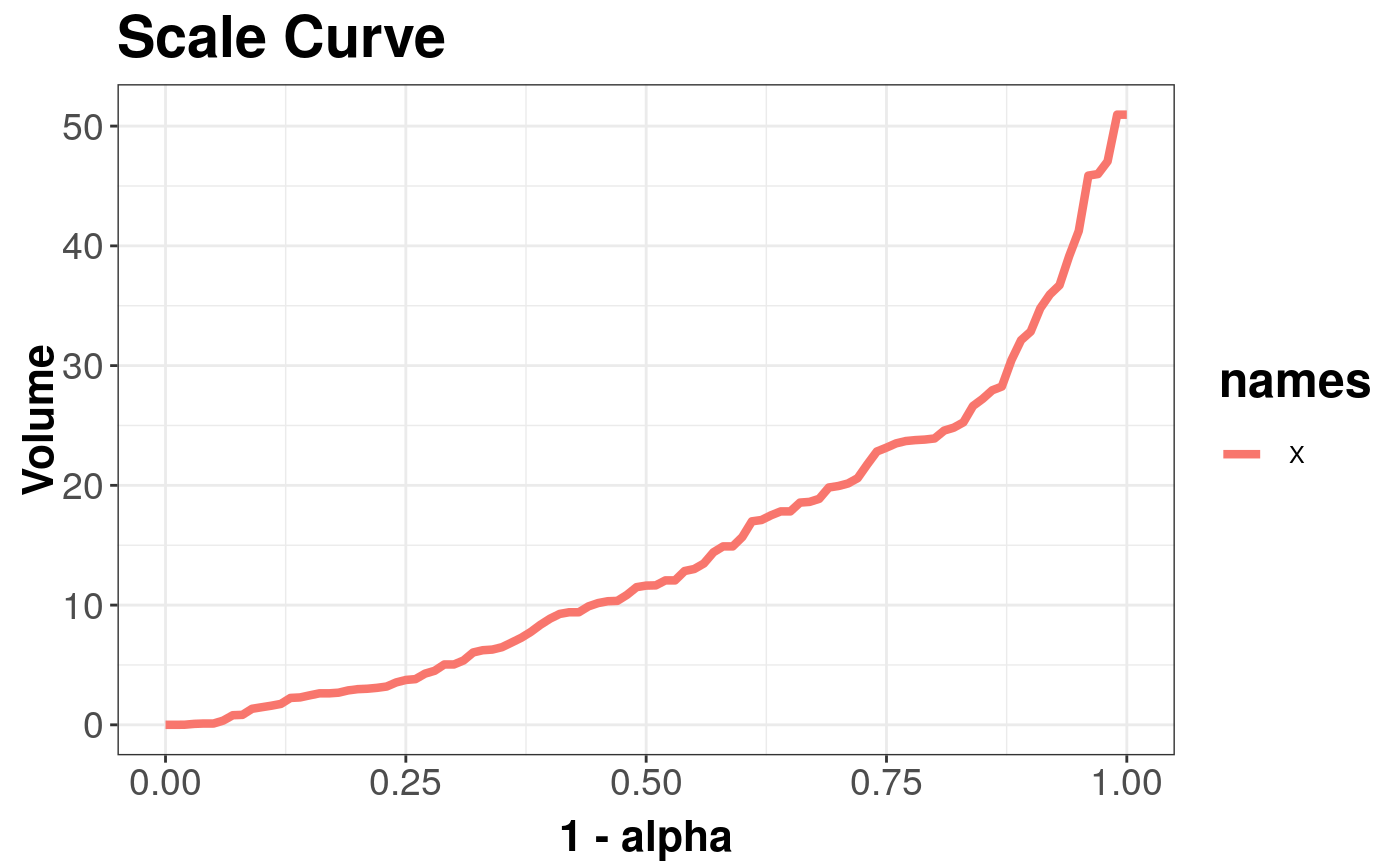Plot Depth curve

plot(x, y, ...)

# S4 method for DDPlot,ANY
plot(x)

# S4 method for DepthCurve,ANY
plot(x)

# S4 method for DepthCurveList,ANY
plot(x)

## Arguments

x object that inherits from DepthCurve class (ScaleCurve or AsymmetryCurve), or DDPlot class. not supported. not supported.

## Examples


x <- mvrnorm(n = 100, mu = c(0, 0), Sigma = 3 * diag(2))
sc <- scaleCurve(x)
plot(sc)